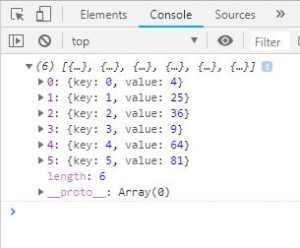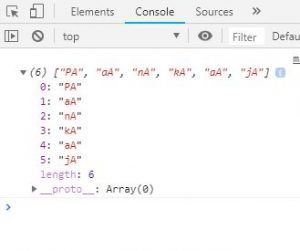Related Articles

# JavaScript | Array map() Method

• Difficulty Level : Basic
• Last Updated : 21 Jul, 2021

The map() method in JavaScript creates an array by calling a specific function on each element present in the parent array. It is a non-mutating method. Generally map() method is used to iterate over an array and calling function on every element of array.
Syntax:

`array.map(function(currentValue, index, arr), thisValue)`

Parameters: This method accepts two parameters as mentioned above and described below:

• function(currentValue, index, arr): It is required parameter and it runs on each element of array. It contains three parameters which are listed below:
• currentValue: It is required parameter and it holds the value of current element.
• index: It is optional parameter and it holds the index of current element.
• arr: It is optional parameter and it holds the array.
• thisValue: It is optional parameter and used to hold the value of passed to the function.

Return Value: It returns a new array and elements of arrays are result of callback function.

Below examples illustrate the use of array map() method in JavaScript:
Example 1: This example use array map() method and return the square of array element.

 ```<``html``>    ``<``head``>``    ``<``title``>``        ``JavaScript Array map() Method``    `````` ` `<``body``>``    ``<``div` `id``=``"root"``>``     ` `    ````    ``<``script``>``     ` `        ``var el = document.getElementById('root');``        ``var arr = [2, 5, 6, 3, 8, 9];``         ` `        ``var newArr = arr.map(function(val, index){``            ``return {key:index, value:val*val};``        ``})``         ` `        ``console.log(newArr)``         ` `        ``el.innerHTML = JSON.stringify(newArr);``    `````` ` `            `

Output:Example 2: This example use array map() method to concatenate character ‘A’ with every character of name.

 ```<``html``>``     ` `<``head``>``    ``<``title``>``        ``JavaScript Array map() Method``    `````` ` `<``body``>``    ``<``div` `id``=``"root"``>`` ` `    ``<``script``>``     ` `        ``var el = document.getElementById('root');``        ``var name = "Pankaj";``         ` `        ``// New array of character and names ``        ``// concatenated with 'A' ``        ``var newName = Array.prototype.map.call(name, function(item) {``            ``return item + 'A';``        ``})``         ` `        ``console.log(newName)``    `````` ` `                    `

Output:Example 3: This example use array map() method to return the square of array elements.

 ```<``html``>``<``head``>``    ``<``title``>``        ``JavaScript Array map() Method``    `````` ` `<``body``>``    ``<``div` `id``=``"root"``>`` ` `    ``<``script``>``        ``var el = document.getElementById('root');``         ` `        ``// Map is being called directly on an array``        ``// Arrow function is used``        ``el.innerHTML = [6, 7, 4, 5].map((val)=>{ ``            ``return val*val;``        ``})``    `````` ` ``

Output:

`36, 49, 16, 25`

JavaScript is best known for web page development but it is also used in a variety of non-browser environments. You can learn JavaScript from the ground up by following this JavaScript Tutorial and JavaScript Examples.

My Personal Notes arrow_drop_up